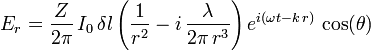# Electric waves

This minimal-math question may have been answered in other thread(s) but they were too mathematical for me.....

Feynman explains clearly, with minimal math, that electromagnetic dipole radiation on axis rapidly approaches zero with distance. But I am wondering if there is not measurable radiation on the axis of a dipole antenna if you are only a few wavelengths away. This would have to be a changing electric field with zero magnetic field - ie, longitudinal electric "waves".

Does exist?

Velocity is c?

Well, the first equation in the wikipedia paragraph you cite does indeed show a non-zero E field varying as a function of time and the dipole's frequency for theta=0:So how does one tease out a velocity (or "propagation delay at two on-axis measurement sites")? And if so, would it not be logical to call these propagation-delayed E-field measurements as some kind of "wave"? Or does a lack of energy transmission imply impossibility of detection [without destroying the field]?

The wording "propagation delay" suggests that you are looking:

• either for the group velocity, corresponding to a wavepacket in a narrow spectral band
• or for the speed of propagation of a brutal wavefront, when a charge is suddenly created on the dipole
• or for any situation in between
• or even for the phase frequency, corresponding to the occurence of maxima or minima of the wave at different places
The full discussion can be quite interresting.
In the second case, there would be an immediate influence at any distance, with the related question: is it possible to create such a charge jump on a dipole? I think this would lead to even wider discussions.

The group velocity would be an easier topic.
You could just analyse the supperposition of two waves with slightly different wavelengths.
The modulation (beats) of the total wave would propagate at the group velocity.
The phase velocity is even simpler, as it is w/k = c, the speed of light.

Last edited:
To be precise, "propagation delay" meant this:
Two on-axis (theta=0) measurement points are selected, one closer to the dipole at r_1 and one farther at r_2. The measured E field at (time=t_1, r=r_1) is E_1 and an almost equal [peak or trough] E_2 is measured at (time=t_2, r=r_2). Then "propagation delay" is t_2 - t_1.

This delay is dt = dr / vp where vp = w/k is the phase velocity.
The factor in front of the phase factor exp(...) doesn't play any role as far as the time when the signal peaks is involved.

A phase velocity of c would certainly be my wild guess, but can you offer any arguement or web site in support? Feynman said nothing about near field velocities. Only that the near field disappears.

Harry,

I was wrong!
The (near field) velocity you are looking for is not exactly the far field phase velocity.
You are considering the delay between two maxima in time at two different locations.
This is, by definition, the phase velocity.
Let us write the electric field in a simple form compatible with the dipole formula:

Er = A(r) exp(i(wt-kr))​
The phase is simply given by:

phase = p(r,t) = arg(Er) = arg(A(r)) + arg(exp(i(wt-kr))) = arg(A(r)) + (wt-kr)​
The term q(r) = arg(A(r)) is a funtion of the position, but not of the time.
Therefore we can write in a more compact way:

phase = q(r) + wt - kr​
To simplify, we can now consider two very close locations and see how a time delay can lead to the same phase:

p(r1,t1) = p(r2,t2)​

q(r1)-q(r2) - k(r1-r2) + w(t1-t2) = 0​
Since r1 and r2 are close, we can assume approximately that

q(r1)-q(r2) = arg(A(r1)/A(r2)) = k' (r1-r2) + neglible terms​
and therefore the condition becomes:

(k'-k)(r1-r2) + w(t1-t2) = 0​
or:

v = (r1-r2)/(t1-t2) = w/(k-k') = w/k * 1/(1-k'/k)​

From this, you can see that when the phase of A changes slowly,
the phase velocity is close to the far field phase velocity, since k'/k << 1 .
Conversly, when k'/k is not negligible, the phase velocity is different from the far field phase velocity.

It is also possible to go forward with the calculation.
Indeed from the dipole formula you get:

q(r1)-q(r2) = arg(1-i/(k.r1)) - arg(1-i/(k.r2))​
from this you can easily get (assuming r1 = r is close to r2):

k'/k = 1/(1+k²r²)​

or finally:

v = w/k * (1 + 1/(k²r²))​

This shows that the near field phase velocity is always larger than the far field phase velocity.
Or in other words, the near field phase velociy is larger than the "speed of light".

Last edited: